# Cole equation of state

The Cole equation of state  is the adiabatic version of the stiffened equation of state. (See Derivation, below.) It has the form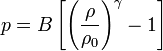$p = B \left[ \left( \frac{\rho}{\rho_0} \right)^\gamma -1 \right]$

In it,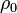$\rho_0$ is a reference density around which the density varies,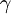$\gamma$ is the adiabatic index, and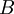$B$ is a pressure parameter.

Usually, the equation is used to model a nearly incompressible system. In this case, the exponent is often set to a value of 7, and$B$ is large, in the following sense. The fluctuations of the density are related to the speed of sound as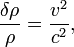$\frac{\delta \rho}{\rho} = \frac{v^2}{c^2} ,$

where$v$ is the largest velocity, and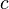$c$ is the speed of sound (the ratio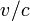$v/c$ is Mach's number). The speed of sound can be seen to be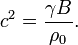$c^2 = \frac{\gamma B}{\rho_0}.$

Therefore, if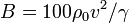$B=100 \rho_0 v^2 / \gamma$, the relative density fluctuations will be about 0.01.

If the fluctuations in the density are indeed small, the equation of state may be approximated by the simpler: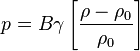$p = B \gamma \left[ \frac{\rho-\rho_0}{\rho_0} \right]$

It is quite common that the name "Tait equation of state" is improperly used for this EOS. This perhaps stems for the classic text by Cole calling this equation a "modified Tait equation" (p. 39).

## Derivation

Let us write the stiffened EOS as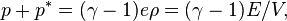$p+ p^* = (\gamma -1) e \rho = (\gamma -1) E / V ,$

where E is the internal energy. In an adiabatic process, the work is the only responsible of a change in internal energy. Hence the first law reads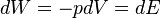$dW= -p dV = dE$

...